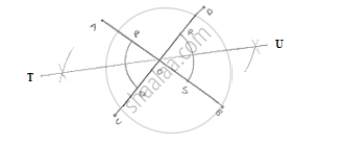# Draw a Pair of Vertically Opposite Angles. Bisect Each of the Two Angles. Verify that the Bisecting Rays Are in the Same Line. - Mathematics

Draw a pair of vertically opposite angles. Bisect each of the two angles. Verify that the bisecting rays are in the same line.

#### SolutionSteps of construction:
1. Draw a pair of vertically opposite angle AOC and DOB
2. With center O and any radius drawn two arcs which intersect OA at P, Q-OB at S and OD at R.
3. With center P and Q and radius more than 1/2PQ draw two arcs which intersect each other at 7.
4. Join to

5. With center R and S radius more than 1/2RS,  draw two arcs which intersect each other at U.
6. Join OU.
∴ TOU is a straight line

Concept: Basic Constructions
Is there an error in this question or solution?

#### APPEARS IN

RD Sharma Mathematics for Class 9
Chapter 16 Constructions
Exercise 16.2 | Q 6 | Page 9

Share